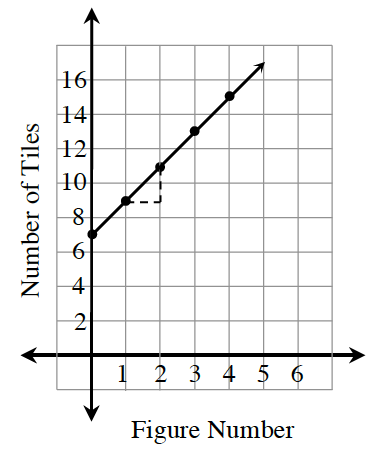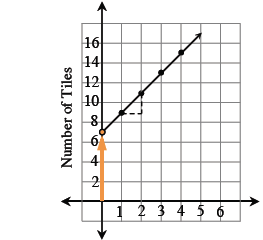Home > ACC7 > Chapter cc34 > Lesson cc34.1.4 > Problem4-38

4-38.Look at the graph at right. What statements can you make about the tile pattern the graph represents? How many tiles are in Figure $0$? Figure $1$? What is the pattern of growth? What is the rule for the pattern?  Homework Help ✎

See graph.Use the same method used to find the number of tiles in
Figure $1$.

Find an equation in the form of $y=mx+b$.

How is the number of tiles changing?

$\frac{\text{change in }y}{\text{change in }x} = \frac{2}{1}$

growth factor $=2$